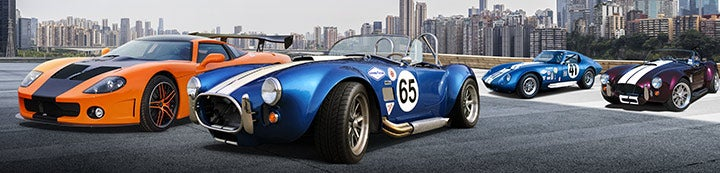1 - 2 of 2 Posts

#### CNIDog2

·
##### Charter Member
Joined
·
251 Posts
Discussion Starter

#### Bob P

·
##### Registered
Joined
·
124 Posts
"The midsize GM caliper I am using on the front has a 2.75" bore caliper which equals 5.94" area according to the chart above. The 1" bore master cylinder I currently have has an area of 0.79. To determine the correct pedal ratio you divide caliper piston area which is 5.94 by master cylinder area which is 0.79"Sq, which equals 7.52. This would mean I would need an effective pedal ratio of 7.52:1 for optimal performance with a 1" bore master cylinder. This is far from the 5.75:1 ratio I have in my car already. So instead of changing the brake pedal ratio I chose to change the bore size of the master cylinder to 1-1/8" (1.12) which as an area of 0.99"Sq. Doing the math with this combination, 5.94 / 0.99 = 6, which equals a 6:1 pedal ratio? So with this combination I am right where I need to be for proper brake pedal travel. "

This makes absolutely no sense. If anything, pedal ratio should be proportional to the inverse of the area ratios. i.e. Large piston area ratio > small pedal ratio.

1 - 2 of 2 Posts